International
Tables for
Crystallography
Volume A
Space-group symmetry
Edited by Th. Hahn

International Tables for Crystallography (2006). Vol. A, ch. 14.2, pp. 870-871

Section 14.2.3.2. Invariant lattice complexes

W. Fischera and E. Kocha*

aInstitut für Mineralogie, Petrologie und Kristallographie, Philipps-Universität, D-35032 Marburg, Germany
Correspondence e-mail:  kochelke@mailer.uni-marburg.de

14.2.3.2. Invariant lattice complexes

| top | pdf |

Invariant lattice complexes in their characteristic Wyckoff position are represented by a capital letter eventually in combination with some superscript. The first column of Table 14.2.3.3gives a complete list of these symbols in alphabetical order. The characteristic Wyckoff positions are shown in column 3. Lattice complexes from different crystal families but with the same coordinate description for their characteristic Wyckoff positions receive the same descriptive symbol. If necessary, the crystal family may be stated explicitly by a small letter (column 2) preceding the lattice-complex symbol: c cubic, t tetragonal, h hexagonal, o orthorhombic, m monoclinic, a anorthic (triclinic).

 Table 14.2.3.3| top | pdf | Descriptive symbols of invariant lattice complexes in their characteristic Wyckoff position
Descriptive symbolCrystal familyCharacteristic Wyckoff position
C o Cmmm a
m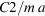D c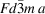o Fddd a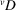t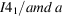E h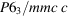F c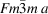o Fmmm a
G h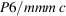I c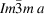t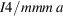o Immm a
J c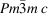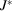c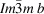M h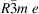N h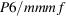P c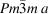h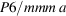t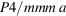o Pmmm a
m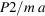a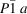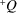h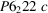R h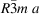S c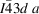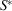c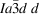T c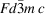o Fddd c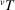t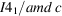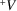c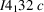c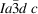W c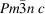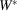c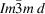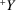c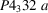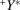c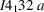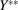c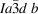Example

D is the descriptive symbol of the invariant cubic lattice complexa as well as of the orthorhombic lattice complex Fddd a. The cubic lattice complex cD contains – among others – the point configurations corresponding to the arrangement of carbon atoms in diamond and of silicon atoms in β-cristobalite. The orthorhombic complex oD is a comprehensive complex of cD. It consists of all those point configurations that may be produced by orthorhombic deformations of the point configurations of cD.

The descriptive symbol of a noncharacteristic Wyckoff position depends on the difference between the coordinate descriptions of the respective characteristic Wyckoff position and the position under consideration. Three cases may be distinguished, which may also occur in combinations.

• (i) The two coordinate descriptions differ by an origin shift. Then, the respective shift vector is added as a prefix to the descriptive symbol of the characteristic Wyckoff position.

Example

The orthorhombic invariant lattice complex C is represented in its characteristic Wyckoff position Cmmm a by the coordinate triplets 000 and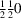. In Ibam a, it is described by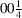,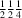and, therefore, receives the descriptive symbol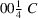.

• (ii) The multiplicity of the Wyckoff position considered is higher than that of the corresponding characteristic position. Then, the coordinate description of this Wyckoff position can be transformed into that of the characteristic position by taking shorter basis vectors. Reduction of all three basis vectors by a factor of 2 is denoted by the subscript 2 on the descriptive symbol. Reduction of one or two basis vectors by a factor of 2 is denoted by one of the subscripts a, b or c or a combination of these. The subscript C means a factor of 3, cc a factor of 4 and Cc a factor of 6.

Examples

The characteristic Wyckoff position of the orthorhombic lattice complex P is Pmmm a with coordinate description 000. It occurs also in Pmma a with coordinate triplets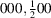, and in Pcca a with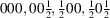. The corresponding descriptive symbols are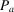and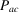, respectively.

• (iii) The coordinate description of a given Wyckoff position is related to that of the characteristic position by inversion or rotation of the coordinate system. Changing the superscript + into − in the descriptive symbol means that the considered Wyckoff position is mapped onto the characteristic position by an inversion through the origin, i.e. both Wyckoff positions are enantiomorphic. A prime preceding the capital letter denotes that a 180° rotation is required.

Examples

 (1)is the descriptive symbol of the invariant lattice complexin its characteristic position. Wyckoff position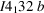with the descriptive symbol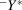belongs to the same lattice complex. The point configurations ofandare enantiomorphic. (2) R is the descriptive symbol of the invariant lattice complex formed by all rhombohedral point lattices. Its characteristic positioncorresponds to the coordinate triplets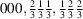. The same lattice complex is symbolized by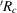in the noncharacteristic position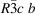with coordinate description 000,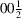,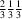,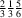,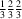,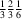.

In noncharacteristic Wyckoff positions, the descriptive symbol P may be replaced by C, I by F (tetragonal system), C by A or B (orthorhombic system), and C by A, B, I or F (monoclinic system). If the lattice complexes of rhombohedral space groups are described in rhombohedral coordinate systems, the symbols R,, M and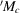of the hexagonal description are replaced by P, I, J and, respectively (preceded by the letter r, if necessary, to distinguish them from the analogous cubic invariant lattice complexes).